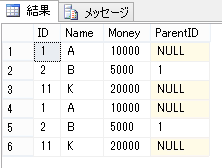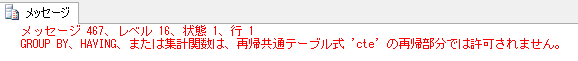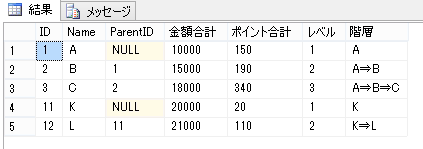# Performing aggregation processing inside recursive processing using common table expressions

Page update date :
Page creation date :

### Sample Data

Use tables with the following hierarchical data and tables with summarized data:

#### Amount hierarchy table

ID (int, PK) Name (nvarchar(50)) Money (bigint) ParentID (int)
1 A 10000 NULL
2 B 5000 1
3 C 3000 2
11 K 20000 NULL
12 L 1000 11

#### Point Table

Point ID (int))
, PKAmount ID (intPoint (bigint)
1 1 100
2 1 50
3 2 40
4 3 10
5 3 60
6 3 80
7 11 20
8 12 50
9 12 40

### How to use common table expressions

I will not write in too much detail because the main topic is not here, but to summarize it simply, a common table expression is like a select expression that you have defined in advance and then you can use a select expression that you have defined without creating multiple of the same description in a later table reference. It may be an image close to the view.

Here's how to use it:

``````-- 共通テーブル式で事前に select 内容を定義する
with [cte] as
(
select *
from [金額階層テーブル]
where [Money] >= 5000
)
select * from [cte]
union all
select * from [cte]
;
``````

#### result### Representation of hierarchical data

I think it's probably this pattern that makes common table expressions so useful. The select expression of the data introduced earlier can be substituted for other things such as views and table variables, so it does not take advantage of much.

Hierarchical representation can be achieved by calling more common table expressions in common table expressions, as in the following SQL:

Points to be careful of when using it are "to combine the select that is the starting point and the select that connects the hierarchical data with union all" and "to unify the size when combining strings".

``````-- 共通テーブル式で階層データをつなげる
with [cte] as
(
select
[ID]
,[Name]
,[ParentID]
,[Money] as [金額合計]
,1 as [レベル]
,cast([Name] as nvarchar(4000)) as [階層]
from [金額階層テーブル]
where [ParentID] is null

union all

select
[子].[ID]
,[子].[Name]
,[子].[ParentID]
,[親].[金額合計] + [子].[Money] as [金額合計]
,[親].[レベル] + 1 as [レベル]
,[親].[階層] + N'⇒' + [子].[Name] as [階層]
from [金額階層テーブル] [子]
inner join [cte] [親]
on [子].[ParentID] = [親].[ID]
)
select *
from [cte]
order by [ID]
;
``````

#### result### Aggregation within the hierarchy processing of common table expressions (error occurred)

I think that there is a case where you want to calculate the aggregated value in the hierarchical processing of the common table expression. Here, we are trying to calculate the total number of points linked to the amount ID.

``````-- 共通テーブル式の階層処理内で集計を使用する (エラー)
with [cte] as
(
select
[ID]
,[Name]
,[ParentID]
,[Money] as [金額合計]
,(
select sum([ポイント])
from [ポイントテーブル]
where [金額ID] = [ID]
) as [ポイント合計]
,1 as [レベル]
,cast([Name] as nvarchar(4000)) as [階層]
from [金額階層テーブル]
where [ParentID] is null

union all

select
[子].[ID]
,[子].[Name]
,[子].[ParentID]
,[親].[金額合計] + [子].[Money] as [金額合計]
,[親].[ポイント合計] + (
select sum([ポイント])
from [ポイントテーブル]
where [金額ID] = [子].[ID]
) as [ポイント合計]
,[親].[レベル] + 1 as [レベル]
,[親].[階層] + N'⇒' + [子].[Name] as [階層]
from [金額階層テーブル] [子]
inner join [cte] [親]
on [子].[ParentID] = [親].[ID]
)
select *
from [cte]
order by [ID]
;
``````

But when I run it, I get the following error:It seems that it is not possible to put grouping-related processing in a recursive common table expression.

### Aggregation in the Hierarchical Processing of Common Table Expressions (Normal Behavior)

Although it is not possible to describe the aggregation process in the recursive common table expression, it can be executed by defining the aggregation processing part as a common table expression.

To write multiple common table expressions, separate the common table expressions with commas.

``````-- 共通テーブル式の階層処理内で集計を使用する (正常)
with [ポイント合計CTE] as
(
select
[金額ID]
,sum([ポイント]) as [ポイント合計]
from [ポイントテーブル]
group by [金額ID]
)
,[cte] as
(
select
[ID]
,[Name]
,[ParentID]
,[Money] as [金額合計]
,(
select [ポイント合計]
from [ポイント合計CTE]
where [金額ID] = [ID]
) as [ポイント合計]
,1 as [レベル]
,cast([Name] as nvarchar(4000)) as [階層]
from [金額階層テーブル]
where [ParentID] is null

union all

select
[子].[ID]
,[子].[Name]
,[子].[ParentID]
,[親].[金額合計] + [子].[Money] as [金額合計]
,[親].[ポイント合計] + (
select [ポイント合計]
from [ポイント合計CTE]
where [金額ID] = [子].[ID]
) as [ポイント合計]
,[親].[レベル] + 1 as [レベル]
,[親].[階層] + N'⇒' + [子].[Name] as [階層]
from [金額階層テーブル] [子]
inner join [cte] [親]
on [子].[ParentID] = [親].[ID]
)
select *
from [cte]
order by [ID]
;
``````

#### result